# Lisp for diagonal lines for selected rectangles

## Recommended PostsA few points to note:

Note that the following filter will exclude closed polylines with linetype generation enabled (since DXF group 70 is bit-coded):

`(ssget (list (cons 0 "LWPOLYLINE")(cons 70 1) (cons 90 4)))`

If an entity name argument is supplied, only the highlighted code will be evaluated (I don't think this is the intention):

```(defun Rectangle-p ( eo fuzz / eo rtn ) ; Grrr
(cond
[highlight]((eq 'ENAME (type eo))
(setq rtn
(vl-every '(lambda (x) (member x '((0 . "LWPOLYLINE") (90 . 4) (70 . 1) (42 . 0))))
(vl-remove-if-not '(lambda (x) (member (car x) '(0 90 70 42))) (entget eo))
)
)
(setq eo (vlax-ename->vla-object eo)); yes go thru the vla check aswell.
)[/highlight]
((eq 'VLA-OBJECT (type eo))
(setq rtn
(and
(= "AcDbPolyline" (vla-get-ObjectName eo))
(vlax-curve-isClosed eo)
(= 4 (vlax-curve-getEndParam eo))
(equal ; opposite sides are equal AB-CD, will accept non-curved arcs/bulges
(apply '- (mapcar '(lambda (x) (vlax-curve-getDistAtParam eo x)) '(1 0)))
(apply '- (mapcar '(lambda (x) (vlax-curve-getDistAtParam eo x)) '(3 2)))
fuzz
)
(equal  ; opposite sides are equal BC-AD, will accept non-curved arcs/bulges
(apply '- (mapcar '(lambda (x) (vlax-curve-getDistAtParam eo x)) '(2 1)))
(apply '- (mapcar '(lambda (x) (vlax-curve-getDistAtParam eo x)) '(4 3)))
fuzz
)
(equal ; diagonals are eq length (not romboid) dist A C = dist B D
(apply 'distance (mapcar '(lambda (x) (vlax-curve-getPointAtParam eo x)) '(1 3)))
(apply 'distance (mapcar '(lambda (x) (vlax-curve-getPointAtParam eo x)) '(2 4)))
fuzz
)
)
)
)
(T nil)
)
rtn
)```

##### Share on other sitesA few points to note:

Note that the following filter will exclude closed polylines with linetype generation enabled (since DXF group 70 is bit-coded):

Thanks Lee,

With the risk to sound stupid, how do you know what bits exactly to use, I mean in the DXF Reference is only described this:

70 Polyline flag (bit-coded); default is 0:

1 = Closed; 128 = Plinegen

But I've seen sometimes you use (70 . 80), and sometimes 3 digits per bit for other GCs.

If an entity name argument is supplied, only the highlighted code will be evaluated (I don't think this is the intention):

I really tried to inspect this issue in VLIDE, stepping thru the evaluations [F8],

but when it gets up to the (entget eo) evaluation, VLIDE opens up some Source #1 page and displays this:

```;;; Copied to window at 10:45 PM 11/23/16

(LAMBDA (X) (MEMBER (CAR X) (QUOTE (0 90 70 42))))

;;; End of text
```

And seems to stop, so I cannot see what evaluates further (is there a way to go thru this? - dummy question #2).

##### Share on other sitesThanks Lee,

With the risk to sound stupid, how do you know what bits exactly to use, I mean in the DXF Reference is only described this:

70 Polyline flag (bit-coded); default is 0:

1 = Closed; 128 = Plinegen

But I've seen sometimes you use (70 . 80), and sometimes 3 digits per bit for other GCs.

DXF group 70 has indeed only two meaningful bit codes for an LWPolyline; perhaps you are thinking of a 2D (heavy) polyline?

I really tried to inspect this issue in VLIDE, stepping thru the evaluations [F8],

but when it gets up to the (entget eo) evaluation, VLIDE opens up some Source #1 page and displays this:

```;;; Copied to window at 10:45 PM 11/23/16

(LAMBDA (X) (MEMBER (CAR X) (QUOTE (0 90 70 42))))

;;; End of text
```

And seems to stop, so I cannot see what evaluates further (is there a way to go thru this? - dummy question #2).

You can declare the lambda expression as a function using the function function:

`(vl-remove-if-not (function (lambda (x) (member (car x) '(0 90 70 42)))) (entget eo))`

But in my opinion, it is easier to use the 'Animate' option offered by the Visual LISP IDE, with an appropriate animation delay (set under Tools > Environment Options > General Options > Diagnostic > Animation delay).

##### Share on other sitesAnother way back to co-ords, did not expect all the zero length lines but it would be easy here (setq co-ordsxy (cons xy co-ordsxy))

to add a check compare new point to the last and if same skip the cons.

##### Share on other sites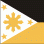Interesting thread. Even for none "lispers" like myself.Good work coders!

I am curious, would it be possible to create just one diagonal in each of the multiple selection of rectangles?

Possibly by stating the corner where the diagonal line originates from, for example "Bottom Left Hand" or "Bottom Right Hand"?

##### Share on other sitesDXF group 70 has indeed only two meaningful bit codes for an LWPolyline; perhaps you are thinking of a 2D (heavy) polyline?

I'll try to work on my drawbacks, maybe I have to do a list where I'm performing badly - oh the irony.

Like bit-handling, ssget filter list operators, wcmatch, dictionaries.

You can declare the lambda expression as a function using the function function:

`(vl-remove-if-not (function (lambda (x) (member (car x) '(0 90 70 42)))) (entget eo))`

But in my opinion, it is easier to use the 'Animate' option offered by the Visual LISP IDE, with an appropriate animation delay (set under Tools > Environment Options > General Options > Diagnostic > Animation delay).

It worked, thats a handy info on apostrophe vs function.

Thank you Lee, you're the best!

##### Share on other sitesManilla wolf if you look at what I posted it draws 2 lines you could at the point of drawing the 1st line zoom in and ask is this correct press or any key and keep or draw the second line. See code below.

The second method a bit more complex would need to work out which point is lower left and use that bearing in mind that a pline can exist clockwise or anticlockwise so the need to compare the two diagonal corner points not 1. You can sort a list of points to find the minimum answer which would be lower left.

This is a starting point, the code could be expanded for do 1, 2, 1 horizontal, 1 vertical, 2 hor & vert, do 4. It does not have the check for zero length lines as per sample dwg.

```; pline co-ords example
; By Alan H
(defun getcoords (ent)
(vlax-safearray->list
(vlax-variant-value
(vlax-get-property
(vlax-ename->vla-object ent)
"Coordinates"
)
)
)
)

(defun co-ords2xy ()
; convert now to a list of xy as co-ords are x y x y x y if 3d x y z x y z
(setq len (length co-ords))
(setq numb (/ len 2)) ; even and odd check required
(setq I 0)
(repeat numb
(setq xy (list (nth i co-ords)(nth (+ I 1) co-ords) ))
; odd (setq xy (list (nth i co-ords)(nth (+ I 1) co-ords)(nth (+ I 2) co-ords) ))
(setq co-ordsxy (cons xy co-ordsxy))
(setq I (+ I 2))
)
)
; program starts here
; change the entsel to a ssget and use repeat for multiple rectangs version 2
(setq co-ords (getcoords (car (entsel "\nplease pick pline"))))
(co-ords2xy) ; list of 2d points making pline

; add a second option here for single line or 2 lines
(command "line" (nth 0 co-ordsxy )(nth 2 co-ordsxy ) "")
(command "zoom" "c" (nth 0 co-ordsxy ) 100)
(setq ans (getstring "is this correct <cr> for ok any other key for no"))
(if (= ans nil)
(princ)
(progn
(command "erase" (entlast) "")
(command "line" (nth 1 co-ordsxy )(nth 3 co-ordsxy ) "")
)
)
```

Edited by BIGAL

##### Share on other sitesThank you BIGAL. I appreciate your contribution.

I comprehend your explanation and logic. You have highlighted that there are many parameters to take into account.

I did get the code to successfully work on a single rectangle. Indeed it worked well.

It may be a little inefficient if adapted to work for multiple rectangles.

I can certainly learn from you code.

Cheers.

##### Share on other sitesIf your doing multiple rectangs as i posted

; change the entsel to a ssget and use repeat for multiple rectangs version 2

Have a go at changing the code

```hint
(setq ss (ssget))
(repeat (setq x (sslength ss))
(setq co-ords (getcoords (ssname ss (setq x (- x 1)))))
(co-ords2xy) ; list of 2d points making pline

..........
```

##### Share on other sitesIf your doing multiple rectangs as i posted

; change the entsel to a ssget and use repeat for multiple rectangs version 2

Have a go at changing the code

```hint
(setq ss (ssget))
(repeat (setq x (sslength ss))
(setq co-ords (getcoords (ssname ss (setq x (- x 1)))))
(co-ords2xy) ; list of 2d points making pline

..........
```

Thanks BIGAL. Unfortunately, it's a little beyond my capabilities.

For reference this is what I tried.

```; pline co-ords example
; By Alan H
(defun getcoords (ent)
(vlax-safearray->list
(vlax-variant-value
(vlax-get-property
(vlax-ename->vla-object ent)
"Coordinates"
)
)
)
)

(defun co-ords2xy ()
; convert now to a list of xy as co-ords are x y x y x y if 3d x y z x y z
(setq len (length co-ords))
(setq numb (/ len 2)) ; even and odd check required
(setq I 0)
(repeat numb
(setq xy (list (nth i co-ords)(nth (+ I 1) co-ords) ))
; odd (setq xy (list (nth i co-ords)(nth (+ I 1) co-ords)(nth (+ I 2) co-ords) ))
(setq co-ordsxy (cons xy co-ordsxy))
(setq I (+ I 2))
)
)
; program starts here
; change the entsel to a ssget and use repeat for multiple rectangs version 2
(setq co-ords (getcoords (car (entsel "\nplease pick pline"))))
(co-ords2xy) ; list of 2d points making pline

; add a second option here for single line or 2 lines
(command "line" (nth 0 co-ordsxy )(nth 2 co-ordsxy ) "")
(command "zoom" "c" (nth 0 co-ordsxy ) 100)
(setq ans (getstring "is this correct <cr> for ok any other key for no"))
(if (= ans nil)
(princ)
(progn
(command "erase" (entlast) "")
(command "line" (nth 1 co-ordsxy )(nth 3 co-ordsxy ) "")
)
)```

##### Share on other sitesManila Wolf said:
Interesting thread. Even for none "lispers" like myself.Good work coders!

Thank youManila Wolf said:
I am curious, would it be possible to create just one diagonal in each of the multiple selection of rectangles?

Possibly by stating the corner where the diagonal line originates from, for example "Bottom Left Hand" or "Bottom Right Hand"?

Consider the following:

```(defun c:rdia ( )
(initget "BLTR TLBR Both")
(rdia (vl-position (cond ((getkword "\nConstruct diagonal [bLTR/TLBR/Both] <Both>: "))("Both")) '(nil "BLTR" "TLBR" "Both")))
(princ)
)
(defun rdia ( bit / ent enx idx lne lst sel zco )
(if
(setq sel
(ssget
'(   (00 . "LWPOLYLINE")
(90 . 4)
(-4 . "&=")   (70 . 1)
(-4 . "<NOT") (-4 . "<>") (42 . 0.0) (-4 . "NOT>")
)
)
)
(repeat (setq idx (sslength sel))
(setq ent (ssname sel (setq idx (1- idx)))
enx (entget ent)
lst (mapcar 'cdr (vl-remove-if-not '(lambda ( x ) (= 10 (car x))) enx))
)
(if (and (equal (distance (car  lst) (cadr  lst)) (distance (caddr lst) (cadddr lst)) 1e-8)
(equal (distance (cadr lst) (caddr lst)) (distance (car   lst) (cadddr lst)) 1e-8)
(equal (distance (car  lst) (caddr lst)) (distance (cadr  lst) (cadddr lst)) 1e-8)
)
(progn
(repeat
(car
(vl-sort-i lst
'(lambda ( a b )
(if (equal (cadr a) (cadr b) 1e-8)
(< (car  a) (car  b))
)
)
)
)
(setq lst (append (cdr lst) (list (car lst))))
)
(setq zco (cdr (assoc 38 enx))
lne
(lambda ( a b )
(entmake
(list
'(0 . "LINE")
(cons 10 (trans (append a (list zco)) ent 0))
(cons 11 (trans (append b (list zco)) ent 0))
)
)
)
)
(if (= 1 (logand 1 bit)) (lne (car  lst) (caddr  lst)))
(if (= 2 (logand 2 bit)) (lne (cadr lst) (cadddr lst)))
)
)
)
)
)
(princ)```

Edited by Lee Mac

##### Share on other sitesConsider the following:

I don't want to sound picky, but from the user's perspective might be more preferable tabbing thru the options with grread.

Similar to:

;; MLeader Arrowhead Toggle - Lee Mac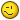##### Share on other sitesThank youConsider the following:

```([color=BLUE]defun[/color] c:rdia ( )
([color=BLUE]initget[/color] [color=MAROON]"BLTR TLBR Both"[/color])
(rdia ([color=BLUE]vl-position[/color] ([color=BLUE]cond[/color] (([color=BLUE]getkword[/color] [color=MAROON]"\nConstruct diagonal [bLTR/TLBR/Both] <Both>: "[/color]))([color=MAROON]"Both"[/color])) '([color=BLUE]nil[/color] [color=MAROON]"BLTR"[/color] [color=MAROON]"TLBR"[/color] [color=MAROON]"Both"[/color])))
([color=BLUE]princ[/color])
)
([color=BLUE]defun[/color] rdia ( bit [color=BLUE]/[/color] ent enx idx lne lst sel zco )
([color=BLUE]if[/color]
([color=BLUE]setq[/color] sel
([color=BLUE]ssget[/color]
'(   (00 . [color=MAROON]"LWPOLYLINE"[/color])
(90 . 4)
(-4 . [color=MAROON]"&="[/color])   (70 . 1)
(-4 . [color=MAROON]"<NOT"[/color]) (-4 . [color=MAROON]"<>"[/color]) (42 . 0.0) (-4 . [color=MAROON]"NOT>"[/color])
)
)
)
([color=BLUE]repeat[/color] ([color=BLUE]setq[/color] idx ([color=BLUE]sslength[/color] sel))
([color=BLUE]setq[/color] ent ([color=BLUE]ssname[/color] sel ([color=BLUE]setq[/color] idx ([color=BLUE]1-[/color] idx)))
enx ([color=BLUE]entget[/color] ent)
lst ([color=BLUE]mapcar[/color] '[color=BLUE]cdr[/color] ([color=BLUE]vl-remove-if-not[/color] '([color=BLUE]lambda[/color] ( x ) ([color=BLUE]=[/color] 10 ([color=BLUE]car[/color] x))) enx))
)
([color=BLUE]if[/color] ([color=BLUE]and[/color] ([color=BLUE]equal[/color] ([color=BLUE]distance[/color] ([color=BLUE]car[/color]  lst) ([color=BLUE]cadr[/color]  lst)) ([color=BLUE]distance[/color] ([color=BLUE]caddr[/color] lst) ([color=BLUE]cadddr[/color] lst)) 1e-
([color=BLUE]equal[/color] ([color=BLUE]distance[/color] ([color=BLUE]cadr[/color] lst) ([color=BLUE]caddr[/color] lst)) ([color=BLUE]distance[/color] ([color=BLUE]car[/color]   lst) ([color=BLUE]cadddr[/color] lst)) 1e-
([color=BLUE]equal[/color] ([color=BLUE]distance[/color] ([color=BLUE]car[/color]  lst) ([color=BLUE]caddr[/color] lst)) ([color=BLUE]distance[/color] ([color=BLUE]cadr[/color]  lst) ([color=BLUE]cadddr[/color] lst)) 1e-
)
([color=BLUE]progn[/color]
([color=BLUE]repeat[/color]
([color=BLUE]car[/color]
([color=BLUE]vl-sort-i[/color] lst
'([color=BLUE]lambda[/color] ( a b )
([color=BLUE]if[/color] ([color=BLUE]equal[/color] ([color=BLUE]cadr[/color] a) ([color=BLUE]cadr[/color] b) 1e-
([color=BLUE]<[/color] ([color=BLUE]car[/color]  a) ([color=BLUE]car[/color]  b))
)
)
)
)
([color=BLUE]setq[/color] lst ([color=BLUE]append[/color] ([color=BLUE]cdr[/color] lst) ([color=BLUE]list[/color] ([color=BLUE]car[/color] lst))))
)
([color=BLUE]setq[/color] zco ([color=BLUE]cdr[/color] ([color=BLUE]assoc[/color] 38 enx))
lne
([color=BLUE]lambda[/color] ( a b )
([color=BLUE]entmake[/color]
([color=BLUE]list[/color]
'(0 . [color=MAROON]"LINE"[/color])
([color=BLUE]cons[/color] 10 ([color=BLUE]trans[/color] ([color=BLUE]append[/color] a ([color=BLUE]list[/color] zco)) ent 0))
([color=BLUE]cons[/color] 11 ([color=BLUE]trans[/color] ([color=BLUE]append[/color] b ([color=BLUE]list[/color] zco)) ent 0))
)
)
)
)
([color=BLUE]if[/color] ([color=BLUE]=[/color] 1 ([color=BLUE]logand[/color] 1 bit)) (lne ([color=BLUE]car[/color]  lst) ([color=BLUE]caddr[/color]  lst)))
([color=BLUE]if[/color] ([color=BLUE]=[/color] 2 ([color=BLUE]logand[/color] 2 bit)) (lne ([color=BLUE]cadr[/color] lst) ([color=BLUE]cadddr[/color] lst)))
)
)
)
)
)
([color=BLUE]princ[/color])
```

Lee, that's amazing.

For my own scenario it's perfect. I do not need to consider rotated rectangles.

I tried the code on over 100 rectangles and it worked very well and very quickly.

Thank you Lee.

(I call you SuperMac. Do you wear your Y Fronts outside your trousers?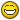)

##### Share on other sitesI don't want to sound picky, but from the user's perspective might be more preferable tabbing thru the options with grread.A nice idea Grrr.

Lee, that's amazing.

For my own scenario it's perfect. I do not need to consider rotated rectangles.

I tried the code on over 100 rectangles and it worked very well and very quickly.

Thank you Lee.

Thanks Manila Wolf, I'm pleased the program is working well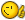(I call you SuperMac. Do you wear your Y Fronts outside your trousers?)

Only when writing code...!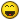##### Share on other sitesThank youConsider the following:

```([color=BLUE]defun[/color] c:rdia ( )
([color=BLUE]initget[/color] [color=MAROON]"BLTR TLBR Both"[/color])
(rdia ([color=BLUE]vl-position[/color] ([color=BLUE]cond[/color] (([color=BLUE]getkword[/color] [color=MAROON]"\nConstruct diagonal [bLTR/TLBR/Both] <Both>: "[/color]))([color=MAROON]"Both"[/color])) '([color=BLUE]nil[/color] [color=MAROON]"BLTR"[/color] [color=MAROON]"TLBR"[/color] [color=MAROON]"Both"[/color])))
([color=BLUE]princ[/color])
)
([color=BLUE]defun[/color] rdia ( bit [color=BLUE]/[/color] ent enx idx lne lst sel zco )
([color=BLUE]if[/color]
([color=BLUE]setq[/color] sel
([color=BLUE]ssget[/color]
'(   (00 . [color=MAROON]"LWPOLYLINE"[/color])
(90 . 4)
(-4 . [color=MAROON]"&="[/color])   (70 . 1)
(-4 . [color=MAROON]"<NOT"[/color]) (-4 . [color=MAROON]"<>"[/color]) (42 . 0.0) (-4 . [color=MAROON]"NOT>"[/color])
)
)
)
([color=BLUE]repeat[/color] ([color=BLUE]setq[/color] idx ([color=BLUE]sslength[/color] sel))
([color=BLUE]setq[/color] ent ([color=BLUE]ssname[/color] sel ([color=BLUE]setq[/color] idx ([color=BLUE]1-[/color] idx)))
enx ([color=BLUE]entget[/color] ent)
lst ([color=BLUE]mapcar[/color] '[color=BLUE]cdr[/color] ([color=BLUE]vl-remove-if-not[/color] '([color=BLUE]lambda[/color] ( x ) ([color=BLUE]=[/color] 10 ([color=BLUE]car[/color] x))) enx))
)
([color=BLUE]if[/color] ([color=BLUE]and[/color] ([color=BLUE]equal[/color] ([color=BLUE]distance[/color] ([color=BLUE]car[/color]  lst) ([color=BLUE]cadr[/color]  lst)) ([color=BLUE]distance[/color] ([color=BLUE]caddr[/color] lst) ([color=BLUE]cadddr[/color] lst)) 1e-
([color=BLUE]equal[/color] ([color=BLUE]distance[/color] ([color=BLUE]cadr[/color] lst) ([color=BLUE]caddr[/color] lst)) ([color=BLUE]distance[/color] ([color=BLUE]car[/color]   lst) ([color=BLUE]cadddr[/color] lst)) 1e-
([color=BLUE]equal[/color] ([color=BLUE]distance[/color] ([color=BLUE]car[/color]  lst) ([color=BLUE]caddr[/color] lst)) ([color=BLUE]distance[/color] ([color=BLUE]cadr[/color]  lst) ([color=BLUE]cadddr[/color] lst)) 1e-
)
([color=BLUE]progn[/color]
([color=BLUE]repeat[/color]
([color=BLUE]car[/color]
([color=BLUE]vl-sort-i[/color] lst
'([color=BLUE]lambda[/color] ( a b )
([color=BLUE]if[/color] ([color=BLUE]equal[/color] ([color=BLUE]cadr[/color] a) ([color=BLUE]cadr[/color] b) 1e-
([color=BLUE]<[/color] ([color=BLUE]car[/color]  a) ([color=BLUE]car[/color]  b))
)
)
)
)
([color=BLUE]setq[/color] lst ([color=BLUE]append[/color] ([color=BLUE]cdr[/color] lst) ([color=BLUE]list[/color] ([color=BLUE]car[/color] lst))))
)
([color=BLUE]setq[/color] zco ([color=BLUE]cdr[/color] ([color=BLUE]assoc[/color] 38 enx))
lne
([color=BLUE]lambda[/color] ( a b )
([color=BLUE]entmake[/color]
([color=BLUE]list[/color]
'(0 . [color=MAROON]"LINE"[/color])
([color=BLUE]cons[/color] 10 ([color=BLUE]trans[/color] ([color=BLUE]append[/color] a ([color=BLUE]list[/color] zco)) ent 0))
([color=BLUE]cons[/color] 11 ([color=BLUE]trans[/color] ([color=BLUE]append[/color] b ([color=BLUE]list[/color] zco)) ent 0))
)
)
)
)
([color=BLUE]if[/color] ([color=BLUE]=[/color] 1 ([color=BLUE]logand[/color] 1 bit)) (lne ([color=BLUE]car[/color]  lst) ([color=BLUE]caddr[/color]  lst)))
([color=BLUE]if[/color] ([color=BLUE]=[/color] 2 ([color=BLUE]logand[/color] 2 bit)) (lne ([color=BLUE]cadr[/color] lst) ([color=BLUE]cadddr[/color] lst)))
)
)
)
)
)
([color=BLUE]princ[/color])
```

what if the rectangles are not perfect, mean to say not exact 90 degree but 89.xx degree and slight variation in length of sides.

##### Share on other siteswhat if the rectangles are not perfect, mean to say not exact 90 degree but 89.xx degree and slight variation in length of sides.

Change 1e-8 fuzz factor to reflect your deviation from correct rectangle shape... I mean change all 1e-8 to some bigger value...

##### Share on other sitesGreat Lisp Mr Lee. Thank you Very Much

##### Share on other sites```(defun c:diago ( / CC CNTR ENTX I IT LINEPR PL_OBJ PT_LST PT_LST_CNT SSPX)

(setq sspx (ssget (list '(0 . "*POLYLINE"))))

(setq i -1)
(repeat (sslength sspx)
(setq pt_lst nil)
(setq it (ssname sspx (setq i (1+ i))))

(setq entx (entget it))
(setq linepr '())
(if (cdr (assoc 6 entx)) (setq linepr (cons (cons 6 (cdr (assoc 6 entx))) linepr)))
(if (cdr (assoc 8 entx)) (setq linepr (cons (cons 8 (cdr (assoc 8 entx))) linepr)))
(if (cdr (assoc 43 entx)) (setq linepr (cons (cons 43 (cdr (assoc 43 entx))) linepr)))
(if (cdr (assoc 62 entx)) (setq linepr (cons (cons 62 (cdr (assoc 62 entx))) linepr)))

(setq	pl_obj (vlax-ename->vla-object it) cc (vla-get-Coordinates pl_obj))

(setq	pt_lst_cnt
(/	(length
(vlax-safearray->list
(vlax-variant-value cc)
)
)
2
)
)

(setq cntr 0)
(repeat pt_lst_cnt
(setq pt_lst
(cons
(append
(vlax-safearray->list
(vlax-variant-value (vla-get-Coordinate pl_obj cntr))
)
(list 0))
pt_lst
)
cntr	 (1+ cntr)
)
)

(if (> (length pt_lst) 3)
(progn
(LWPoly (list (nth 0 pt_lst)(nth 2 pt_lst)) linepr)
(LWPoly (list (nth 1 pt_lst)(nth 3 pt_lst)) linepr)
)
)

);repeat
)

;;;;; By Lee MAC
(defun LWPoly (lst lprx)
(setq ll (list
(cons 0 "LWPOLYLINE")
(cons 100 "AcDbEntity")
(cons 100 "AcDbPolyline")
(cons 90 (length lst))
))

(setq all (append ll lprx ))
(entmakex (append all (mapcar (function (lambda (p) (cons 10 p))) lst)))
)```

•1

##### Share on other sitesOn 12/28/2017 at 4:33 PM, handasa said:
```
(defun c:diago ( / CC CNTR ENTX I IT LINEPR PL_OBJ PT_LST PT_LST_CNT SSPX)

(setq sspx (ssget (list '(0 . "*POLYLINE"))))

(setq i -1)
(repeat (sslength sspx)
(setq pt_lst nil)
(setq it (ssname sspx (setq i (1+ i))))

(setq entx (entget it))
(setq linepr '())
(if (cdr (assoc 6 entx)) (setq linepr (cons (cons 6 (cdr (assoc 6 entx))) linepr)))
(if (cdr (assoc 8 entx)) (setq linepr (cons (cons 8 (cdr (assoc 8 entx))) linepr)))
(if (cdr (assoc 43 entx)) (setq linepr (cons (cons 43 (cdr (assoc 43 entx))) linepr)))
(if (cdr (assoc 62 entx)) (setq linepr (cons (cons 62 (cdr (assoc 62 entx))) linepr)))

(setq	pl_obj (vlax-ename->vla-object it) cc (vla-get-Coordinates pl_obj))

(setq	pt_lst_cnt
(/	(length
(vlax-safearray->list
(vlax-variant-value cc)
)
)
2
)
)

(setq cntr 0)
(repeat pt_lst_cnt
(setq pt_lst
(cons
(append
(vlax-safearray->list
(vlax-variant-value (vla-get-Coordinate pl_obj cntr))
)
(list 0))
pt_lst
)
cntr	 (1+ cntr)
)
)

(if (> (length pt_lst) 3)
(progn
(LWPoly (list (nth 0 pt_lst)(nth 2 pt_lst)) linepr)
(LWPoly (list (nth 1 pt_lst)(nth 3 pt_lst)) linepr)
)
)

);repeat
)

;;;;; By Lee MAC
(defun LWPoly (lst lprx)
(setq ll (list
(cons 0 "LWPOLYLINE")
(cons 100 "AcDbEntity")
(cons 100 "AcDbPolyline")
(cons 90 (length lst))
))

(setq all (append ll lprx ))
(entmakex (append all (mapcar (function (lambda (p) (cons 10 p))) lst)))
)```

I like this.It simple and perfect to use

## Join the conversation

You can post now and register later. If you have an account, sign in now to post with your account.
Note: Your post will require moderator approval before it will be visible.Reply to this topic...

×   Pasted as rich text.   Paste as plain text instead

Only 75 emoji are allowed.